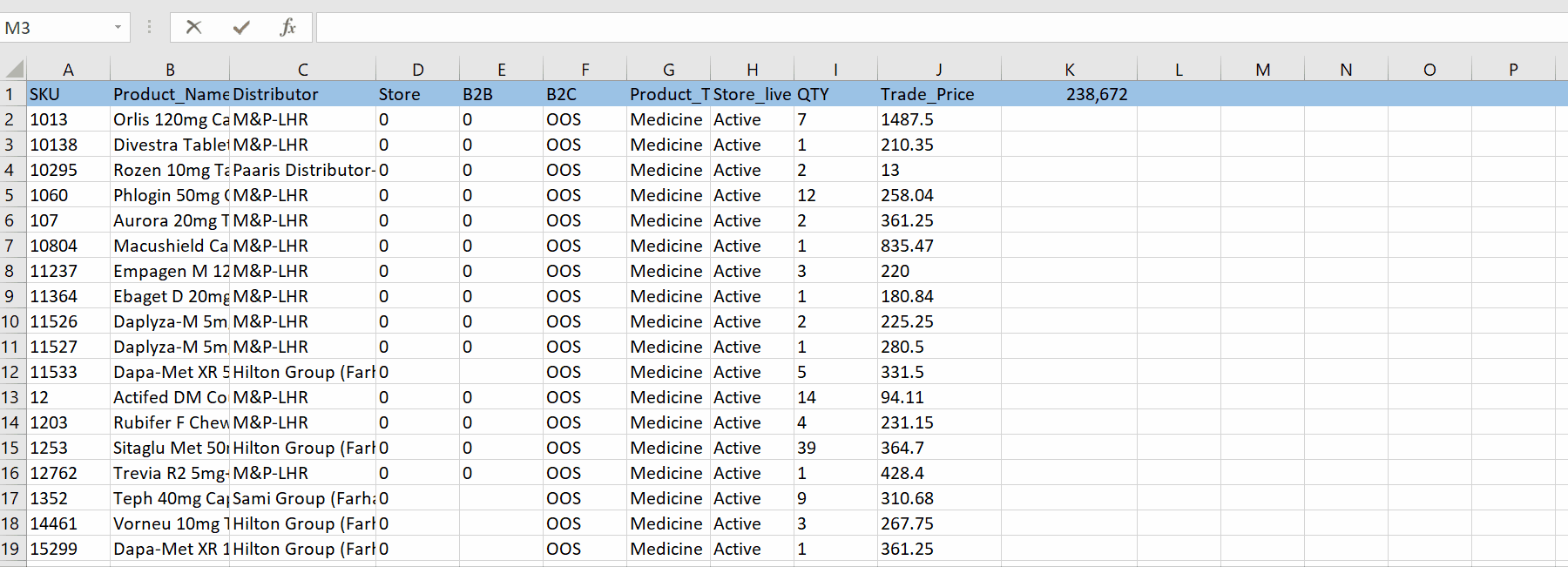# How to use SUMIFS in multiple columns

In this tutorial, we will learn how to use SUMIFS in Excel.

Excel provides a formula which is given below;

=SUMIFS(sum_range, criteria_range1, criteria1, [criteria_range2, criteria2], …)

Sum_range : The range of cells to sum.

Criteria_range1 : The range that is tested using Criteria1.

(Criteria_range1 and Criteria1 set up a search pair whereby a range is searched for specific criteria. Once items in the range are found, their corresponding values in Sum_range are added).

Criteria1 : The criteria that defines which cells in Criteria_range1 will be added.

Criteria_range2, criteria2,…    (optional)

Note : Additional ranges and their associated criteria. You can enter up to 127 range/criteria pairs.

In the given example of sample data in which we will calculate the out of stock(OOS) count for a specific distributor namely M&P-LHR in a specific column.

Below are the steps to follow.Microsoft Excel is one of the best numeric data calculation software produced by Microsoft and it gained popularity around the globe due to its agility , accuracy , smoothness, and sharpness in calculations . Data calculation is one of the key features in Excel. Sometimes we need to calculate SUM from different columns with specific conditions. To calculate, Excel provides a built-in formula for it.

### Step 1 – Select the cell and apply formula– Select the cell and type the formula.

– Select the sum range , in this case we selected column I the quantity  that we want to calculate

– Select the criteria of range , for this case we selected column C the distributor.

– Select the criteria that defines the cell. In this case we selected M&P-LHR.

– Select the criteria of the second range , for this case we selected column F the B2C.

– Select the second criteria that defines the cell. In this case we selected OOS.

– As soon as you press the enter key. Results will be provided.
Above is an animation as an example.

Hence, we have successfully got the sum (485) of total out of stock(OOS) items in the B2C segment of a specific distributor.